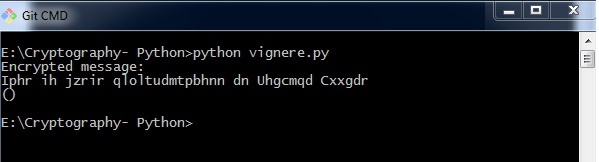# Implementing Vignere Cipher

In this chapter, let us understand how to implement Vignere cipher. Consider the text This is basic implementation of Vignere Cipher is to be encoded and the key used is PIZZA.

## Code

You can use the following code to implement a Vignere cipher in Python −

import pyperclip

LETTERS = 'ABCDEFGHIJKLMNOPQRSTUVWXYZ'
def main():
myMessage = "This is basic implementation of Vignere Cipher"
myKey = 'PIZZA'
myMode = 'encrypt'

if myMode == 'encrypt':
translated = encryptMessage(myKey, myMessage)
elif myMode == 'decrypt':
translated = decryptMessage(myKey, myMessage)

print('%sed message:' % (myMode.title()))
print(translated)
print()
def encryptMessage(key, message):
return translateMessage(key, message, 'encrypt')
def decryptMessage(key, message):
return translateMessage(key, message, 'decrypt')
def translateMessage(key, message, mode):
translated = [] # stores the encrypted/decrypted message string
keyIndex = 0
key = key.upper()

for symbol in message:
num = LETTERS.find(symbol.upper())
if num != -1:
if mode == 'encrypt':
num += LETTERS.find(key[keyIndex])
elif mode == 'decrypt':
num -= LETTERS.find(key[keyIndex])
num %= len(LETTERS)

if symbol.isupper():
translated.append(LETTERS[num])
elif symbol.islower():
translated.append(LETTERS[num].lower())
keyIndex += 1

if keyIndex == len(key):
keyIndex = 0
else:
translated.append(symbol)
return ''.join(translated)
if __name__ == '__main__':
main()


## Output

You can observe the following output when you implement the code given above −The possible combinations of hacking the Vignere cipher is next to impossible. Hence, it is considered as a secure encryption mode.